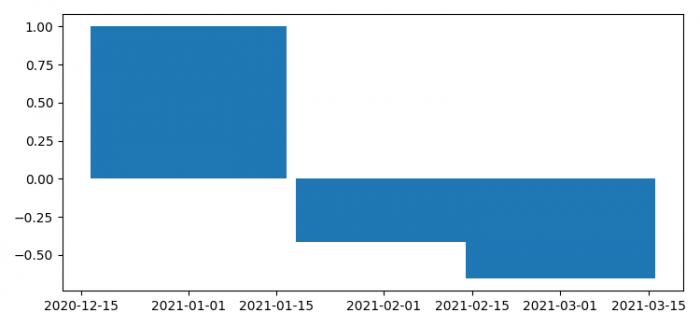# Controlling the width of bars in Matplotlib with per-month data

To control the width of bars in matplotlib with per-month data, we can take the following steps −

• Set the figure size and adjust the padding between and around the subplots
• Make a list of dates, x and y, using numpy.
• Plot the bar with x and y data points, with per-month data.
• To display the figure, use show() method.

## Example

import numpy as np
import datetime
from matplotlib import pyplot as plt

plt.rcParams["figure.figsize"] = [7.50, 3.50]
plt.rcParams["figure.autolayout"] = True

x = [datetime.datetime(2021, 1, 1, 0, 0),
datetime.datetime(2021, 2, 1, 0, 0),
datetime.datetime(2021, 3, 1, 0, 0),
]

y = np.cos(np.arange(3) * 2)
plt.bar(x, y, width=[(x[j+1]-x[j]).days for j in range(len(x)-1)] + )

plt.show()

## Output